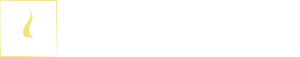# Absorption of Radiation - Fundamentals of Energy AbsorptionB.Sc (Hons, USJ) (Polymer Science and Technology, Chemistry, Physics)
Categories
Last Updated On : January 20, 2023
Published Date : June 17, 2022

Everything around us can be seen because of the absorption of radiation (absorption of energy) and emission of energy (Electromagnetic radiation). Different types of molecules can absorb energy with different wavelengths.

There are two laws have been introduced to explain the energy absorption by molecules.

## 1. Grotthuss Draper law

When the light is fall on the molecules, only a fraction of the energy will be absorbed by the molecules. Due to the absorbed energy, the molecule gets in an excitation state. Only that light absorbed by the molecule brings photochemical changes in the molecule.

The excited molecules can release the absorbed energy and get into the ground state again. This process is called irradiation. The energy is released as the wavelengths of the electromagnetic spectrum. In photochemical reaction, we consider the “Quantum yield”

The emitted photon is measured by using fluorescence spectroscopy.

## 2. Stark–Einstein law

For each quantum of radiation, one molecule of substance is excited.

When a range of wavelengths passes through the substance, some of the wavelengths will be absorbed by the substance molecules and some wavelengths just pass through the substance. Wavelengths can also reflect or refract from the substance. However, the emitted quantum is less.

Molecules of the excited state have high energy. So, they are very unstable. Due to the absorbance of energy, intramolecular bonds will be weaker, and the electrons will go to the antibonding orbitals. Therefore, excited molecules are highly reactive.

Absorption of radiation can be described by using the “Beer-Lambert law”. When the radiation passes through a solution, some of the radiation is absorbed by the solution. Therefore, the intensity of the transmitted radiation is always lower than the incident radiation.

The absorbance is given by,

Where,

• A = absorbance
• I0 = intensity of the incident radiation
• I1 = intensity of the transmitted radiation
• ε = molar absorption coefficient
• C = concentration of the solution
• l = path length

After absorbing radiation, molecules get into the excited state. The excited molecules are highly unstable. So, they tend to release the absorbed energy and become the ground state again. The absorption and emission of energy can be described by using the Jablonski diagram.

Excited electrons are coming back to the ground state by releasing energy. Energy releasing can be radiative or non-radiative. Florescence and phosphorescence are radiative while vibrational relaxation and intersystem crossing are non-radiative.

Furthermore, the excited molecules are vibrating due to their high energy. So, they collide with each other and release energy. This is also a non-radiative process. But it is an inter-molecular phenomenon.

According to the molecular orbital theory, following transitions can be taken place between bonding and antibonding orbitals.

• n to π*
• π to π*
• σ to π*
• σ to σ*

These electron transitions are taken place in the UV-visible range. If IR is applied, electrons cannot be made any transitions because the energy is not enough.

## The intensity of the transmitted radiation

If there are high electron transitions, the intensity of the transmitted radiation is high. Intensity is governed by oscillator factor (f).

Where,

• I0 = intensity of the incident radiation
• I = intensity of the transmitted radiation

The maximum value for the oscillator factor is 1 and the minimum value is 0. The oscillator factor depends on another four factors.

### Electron spin (Ps)

Electron spin is one of the properties of electrons and gives magnetic properties to the atom. To change the direction of spin, electrons should be absorbed energy. The change of spin makes the transition of electrons between the singlet state to the triplet state. Transitions between singlet states result in fluorescence and transitions between singlet-triplet states result in phosphorescence.

The energy gap between singlet to triplet is lower than the singlet – singlet. That is why fluorescence has a much higher intensity than phosphorescence. If there are more singlet-triplet transitions, the intensity of emitted radiation is decreased.

Theoretically, changing the spin does not happen; it is forbidden. That means the transitions between singlet state to triplet state are forbidden. But phosphorescence also happens. But it is theoretically forbidden.

### Orbital symmetry (PO)

Electron transitions are taken place only between the orbitals which are in the same plane. If there are electrons in the same plane, a high number of transitions are taken place. So, the intensity will increase. There are no transitions between orbitals that are perpendicular to each other.

### Parity (Pp)

Parity describes the symmetry of orbitals. Orbitals with symmetry are called gerade (G) or even and orbitals with no symmetry are Ungeade (U) or odd.  π bonding orbitals are ungerade and to π* antibonding orbitals are gerade.  When transition of electrons gerade to ungerade or ungerade to gerade are allowed.

### Momentum (Pm)

Change of spin makes the change of angular momentum. Large change of momentum results in low intensity. Therefore, a large change of momentum is forbidden.## References and Attributes

### Figures:

The cover image was created using an image by Uwee Westphal from Pixabay